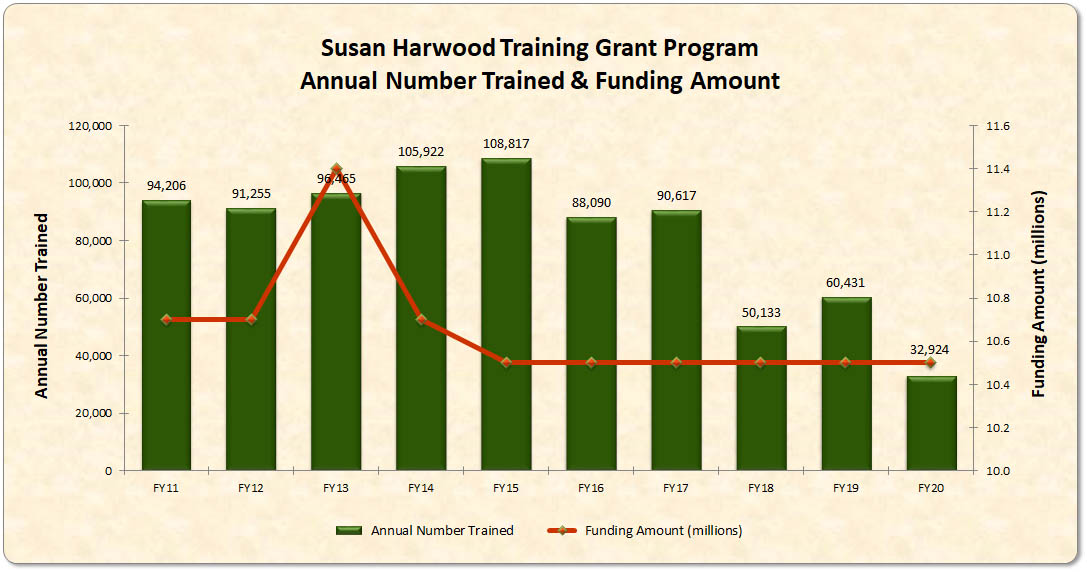Susan Harwood Training Grant Program

### Program Statistics#### Number of trainees in the last 5 years = 322,195 Number of trainees in the last 10 years = 1,510,534

TEXT VERSION OF CHART:

Title:
Susan Harwood Training Grant Program
Type:
Vertical Bar and Horizontal Line Graph
Chart Elements:
20 - one bar and one point for each fiscal year from 2011 to 2020 showing the number trained and the amount awarded per fiscal year

Values:

• FY 20 =
• Annual Number Trained = 32,924; Awarded (\$, millions) = 10.5
• FY 19 =
• Annual Number Trained = 60,431; Awarded (\$, millions) = 10.5
• FY 18 =
• Annual Number Trained = 50,133; Awarded (\$, millions) = 10.5
• FY 17 =
• Annual Number Trained = 90,617; Awarded (\$, millions) = 10.5
• FY 16 =
• Annual Number Trained = 88,090; Awarded (\$, millions) = 10.5
• FY 15 =
• Annual Number Trained = 108,817; Awarded (\$, millions) = 10.5
• FY 14 =
• Annual Number Trained = 105,922; Awarded (\$, millions) = 10.7
• FY 13 =
• Annual Number Trained = 96,465; Awarded (\$, millions) = 11.4
• FY 12 =
• Annual Number Trained = 91,255; Awarded (\$, millions) = 10.7
• FY 11 =
• Annual Number Trained = 94,206; Awarded (\$, millions) = 10.7
• FY 10 =
• Annual Number Trained = 65,732; Awarded (\$, millions) =10.7